# Non Verbal Reasoning - Water Images

### Exercise :: Water Images - Section 2

In each of the following questions, choose the water image of the Fig.(X) from amongst the four alternatives (1), (2), (3) and (4) given along with it.

16.

Choose the correct water image of the given figure (X) from amongst the four alternatives.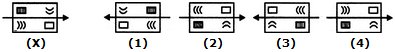A. 1 B. 2 C. 3 D. 4

Explanation:

No answer description available for this question. Let us discuss.

17.

Choose the correct water image of the given figure (X) from amongst the four alternatives.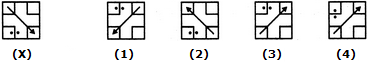A. 1 B. 2 C. 3 D. 4

Explanation:

No answer description available for this question. Let us discuss.

18.

Choose the correct water image of the given figure (X) from amongst the four alternatives.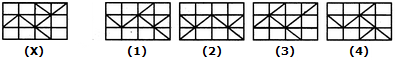A. 1 B. 2 C. 3 D. 4

Explanation:

No answer description available for this question. Let us discuss.

19.

Choose the correct water image of the given figure (X) from amongst the four alternatives.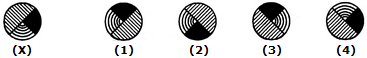A. 1 B. 2 C. 3 D. 4

Explanation:

No answer description available for this question. Let us discuss.

20.

Choose the correct water image of the given figure (X) from amongst the four alternatives.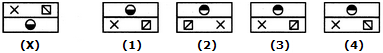A. 1 B. 2 C. 3 D. 4